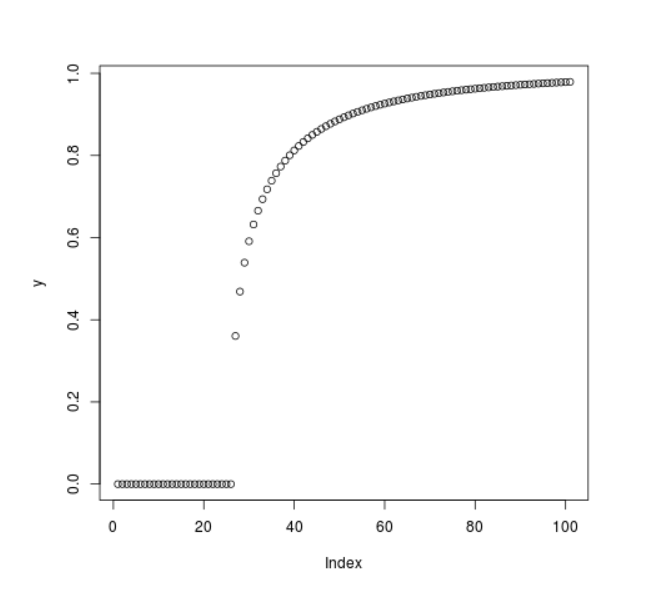Open in App
Not now

# Compute the Value of Cumulative Weibull Density in R Programming – pweibull() Function

• Last Updated : 25 Jun, 2020

`pweibull()` function in R Language is used to compute the value of Cumulative Distribution Function for Weibull Distribution. It also plots a density graph for Cumulative Weibull Function.

Syntax: pweibull(x, shape)

Parameters:
x: Numeric Vector
shape: Shape Parameter

Example 1:

 `# R Program to compute``# Cumulative Weibull Density`` ` `# Creating a sequence of x-values``x <``-` `seq(``-``5``, ``15``, by ``=` `1``)`` ` `# Calling pweibull() Function``y <``-` `pweibull(x, shape ``=` `0.2``)``y`

Output:

```  0.0000000 0.0000000 0.0000000 0.0000000 0.0000000 0.0000000 0.6321206
 0.6829508 0.7122695 0.7327332 0.7483534 0.7609229 0.7713981 0.7803493
 0.7881434 0.7950303 0.8011877 0.8067464 0.8118052 0.8164408 0.8207138
```

Example 2:

 `# R Program to compute``# Cumulative Weibull Density`` ` `# Creating a sequence of x-values``x <``-` `seq(``-``5``, ``15``, by ``=` `0.2``)`` ` `# Calling pweibull() Function``y <``-` `pweibull(x, shape ``=` `0.5``)`` ` `# Plot a graph``plot(y)`

Output:My Personal Notes arrow_drop_up